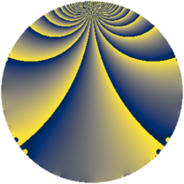# Properties

 Label 770.2.rLevel $770$ Weight $2$ Character orbit 770.r Rep. character $\chi_{770}(529,\cdot)$ Character field $\Q(\zeta_{6})$ Dimension $80$ Newform subspaces $2$ Sturm bound $288$ Trace bound $6$

# Related objects

## Defining parameters

 Level: $$N$$ $$=$$ $$770 = 2 \cdot 5 \cdot 7 \cdot 11$$ Weight: $$k$$ $$=$$ $$2$$ Character orbit: $$[\chi]$$ $$=$$ 770.r (of order $$6$$ and degree $$2$$) Character conductor: $$\operatorname{cond}(\chi)$$ $$=$$ $$35$$ Character field: $$\Q(\zeta_{6})$$ Newform subspaces: $$2$$ Sturm bound: $$288$$ Trace bound: $$6$$ Distinguishing $$T_p$$: $$3$$

## Dimensions

The following table gives the dimensions of various subspaces of $$M_{2}(770, [\chi])$$.

Total New Old
Modular forms 304 80 224
Cusp forms 272 80 192
Eisenstein series 32 0 32

## Trace form

 $$80q + 40q^{4} + 8q^{6} + 44q^{9} + O(q^{10})$$ $$80q + 40q^{4} + 8q^{6} + 44q^{9} + 8q^{14} + 40q^{15} - 40q^{16} + 16q^{19} - 12q^{21} + 4q^{24} - 8q^{25} - 24q^{26} - 56q^{29} - 20q^{30} + 24q^{31} - 32q^{34} - 20q^{35} + 88q^{36} + 32q^{39} - 24q^{41} - 12q^{45} - 12q^{46} - 36q^{49} + 32q^{50} + 24q^{51} + 4q^{54} + 4q^{56} + 16q^{59} + 20q^{60} - 28q^{61} - 80q^{64} + 12q^{65} - 16q^{66} + 56q^{69} - 16q^{70} - 48q^{71} + 24q^{74} - 28q^{75} + 32q^{76} + 24q^{79} - 72q^{81} + 48q^{84} + 48q^{85} + 4q^{86} + 68q^{89} - 24q^{90} - 64q^{91} - 56q^{94} + 4q^{95} - 4q^{96} + O(q^{100})$$

## Decomposition of $$S_{2}^{\mathrm{new}}(770, [\chi])$$ into newform subspaces

Label Dim. $$A$$ Field CM Traces $q$-expansion
$$a_2$$ $$a_3$$ $$a_5$$ $$a_7$$
770.2.r.a $$40$$ $$6.148$$ None $$0$$ $$0$$ $$0$$ $$0$$
770.2.r.b $$40$$ $$6.148$$ None $$0$$ $$0$$ $$0$$ $$0$$

## Decomposition of $$S_{2}^{\mathrm{old}}(770, [\chi])$$ into lower level spaces

$$S_{2}^{\mathrm{old}}(770, [\chi]) \cong$$ $$S_{2}^{\mathrm{new}}(35, [\chi])$$$$^{\oplus 4}$$$$\oplus$$$$S_{2}^{\mathrm{new}}(70, [\chi])$$$$^{\oplus 2}$$$$\oplus$$$$S_{2}^{\mathrm{new}}(385, [\chi])$$$$^{\oplus 2}$$